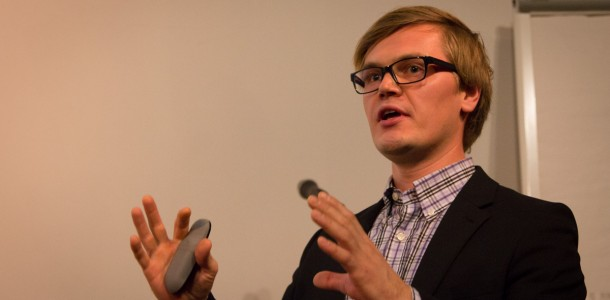###### PhD thesis defense: Otto Nissfolk

September 30, 2016 Andreas Lundell Events, News

The public defence of Otto Nissfolk’s PhD thesis, “Binary Quadratic Optimization” will take place on Friday September 30, 2016, 12 PM in Auditorium Salin, Axelia II, Biskopsgatan 8, Åbo.

Introduction:

Optimization is an important activity in decision making, in analyzing and in improving production in a factory. In mathematical terms, an optimization problem is the problem of finding the best solution out of all feasible solutions. An optimization problem consists of an objective function, variables and constraints. The objective function is a mathematical function expressing what we want to maximize or minimize. For example, in manufacturing we may want to maximize the profits or minimize the cost of production. The variables can express how much resources are needed or the time spent in various production steps. Binary variables can be decision variables expressing whether a factory should be built at a location or not. The constraints are functions that define the boundaries for the variables, e.g. the amount of resources used cannot exceed the available resources.

In order to solve a problem to optimality, the problem often needs to be convex, that means that a local minimum is also the global one. If the problem is not convex it can be convexified. In this thesis, we work with quadratic problems and in order to obtain a convex problem the quadratic matrix needs to be positive-semidefinite, that is that all eigenvalues of the matrix are in the positive half-space of the complex plane. If the optimal solution cannot be obtained we can still obtain indications on how good the solution is with a upper bound (UB) and a lower bound (LB). A upper bound is a solution that fulfills all constraints and is therefore a feasible solution. The lower bound is a relaxed solution where some of the constraints may not be active. When the upper and the lower bound are alike, the problem is solved to optimality.

A linear program (LP) is a problem formulation including only continuous variables and linear constraints. When introducing integer variables, the problem goes from LP to mixed integer linear programming (MILP). If there are quadratic terms in the objective function, the problem is called quadratic program (QP) and if there are as well quadratic constraints, it is a quadratically constrained quadratic program (QCQP). One can use heuristic methods in order to obtain good solutions in a short amount of time. However, the solution is neither guaranteed to be the optimal solution, nor can any bounds for the solution be obtained.